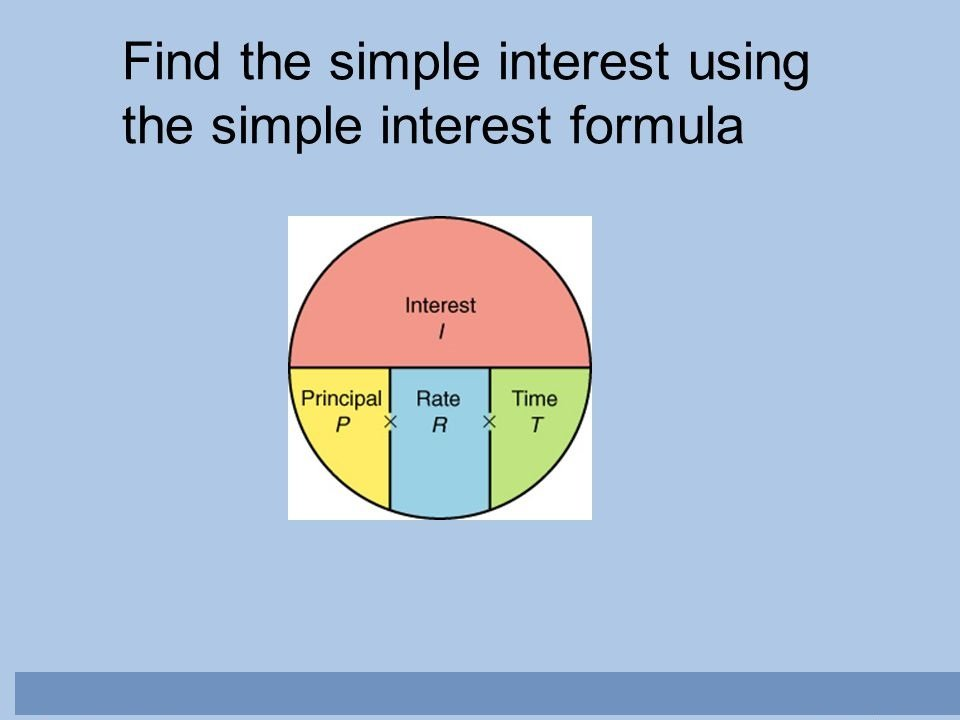# Simple Interest Formula – Explanation with a Solved Example

Simple interest is a term that you must have heard quite frequently. It is one of the most important financial terms. You can understand simple interest as the amount that you pay on the principal over a particular duration of time (months or years). This interest is a fixed percentage of the principal amount that you borrowed or lent.The simple interest formula is as follows:

Simple Interest (S.I.) = P * R * T/100

Here, P is the principal, R is the rate of interest and T is the time a person takes to clear his/her loan.

Now, what do we understand by this term, and where do we find its usage in our life? Well, here is an example for the same:

Please note that interest is the cost of borrowing money from a person. You can consider it as the borrowing fee that you pay along with the borrowed money to the lender. So, here we will look at one example of simple interest and solve it in a simpler manner to understand how taking and paying the loan works between your friends, relatives, banks, or any money-lending organization you take a loan from. So, let’s get started.

### Simple Interest Formula with a Solved Example

A person named Rina wanted to purchase a gift worth Rs. 500. However, she had only 100 Rs in her piggy bank. Now, if she takes a loan of Rs. 400 from the shopkeeper at an 8% rate of interest and promises to clear this loan in 2 years. What can be the simple interest for this principal? Well, for this, we can put the values from our example into the above Simple Interest Formula, so here we go:

Here,

P = 400,

R = 8%, and

T = 2 years

So, SI = 400 * 8 * 2/100

On solving, we get 64 as our simple interest or the borrowing fee for the gift. Hence, Rina pays the simple interest of 64 to clear her loan of Rs. 400.

### Example on the Compound Interest

On Diwali, Rehan booked the HD T.V. at the Samsung showroom at a loan of Rs. 30, 000 from his own finance company. He promised to pay this amount after three years. The company charges compound interest at the rate of 10% per annum for the same. Suddenly, he got shocked that his company announced the rate of interest as 20% per annum for the last year of the loan period. Now, how much extra amount does Rehan have to pay because of the announcement of a hike in the rate of interest?

So, we have the following formula:

C.I. = P (1 + R/100)n

Here, P and R are the same things we discussed above, and n = numbers of years after which Rehan promised to pay the amount.

The amount he was supposed to pay was:

C.I. = 30, 000 (1 + 10/100)3

= 399,30….(1)

Now, the amount paid for the first two years:

C.I. = 30, 000 (1 + 10/100)2

On solving, we get, C.I. = 3,630

In the last year, R = 25%, so

C.I. = 30, 000 (1 + 20/100)

= 36,000….(2)

Therefore, the extra amount Rehan had to pay is 39930 – 36000 = Rs. 3930.

### Difference between Simple Interest and Compound Interest

The basic difference between these two terms is, that simple interest is the simpler form of interest a person takes and clears it out soon. However, compound interest is like a cycle of principal, and the amount a person keeps on paying and it becomes very hard to clear this type of loan.

You can see that the amount that you have paid in one year gets added to your previous interests and the cycle of paying continues till you clear your loan.

Basically, what happens here is, simple interest on the principal amount over a certain period (or years) is a fixed percentage of the principal amount that you borrowed or lent.

However, compound interest accrues and gets added to the accumulated interest of previous periods, so borrowers must pay interest on interest as well as principal.#### sachin

He is a Blogger, Tech Geek, SEO Expert, and Designer. Loves to buy books online, read and write about Technology, Gadgets and Gaming. you can connect with him on Facebook | Linkedin | mail: srupnar85@gmail.com

sachin has 304 posts and counting. See all posts by sachin